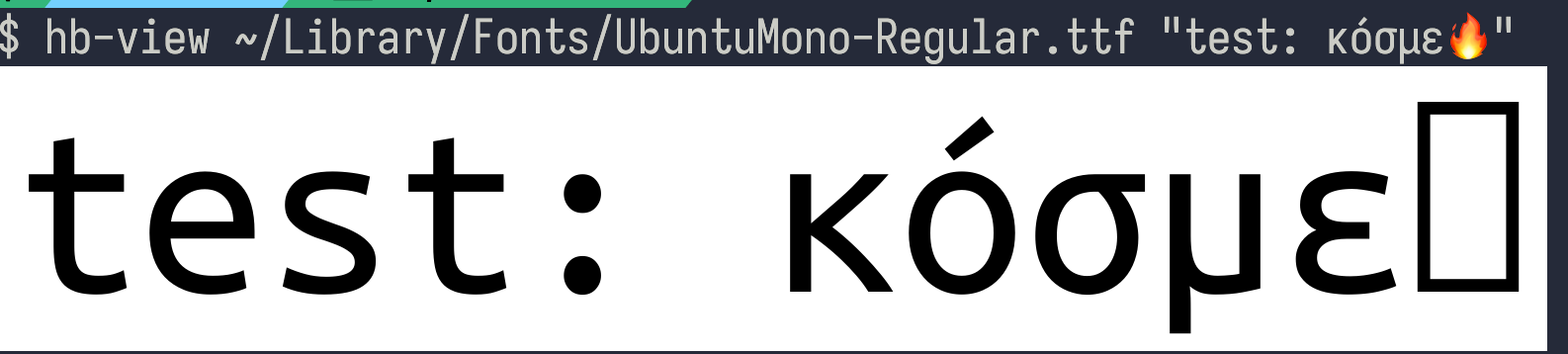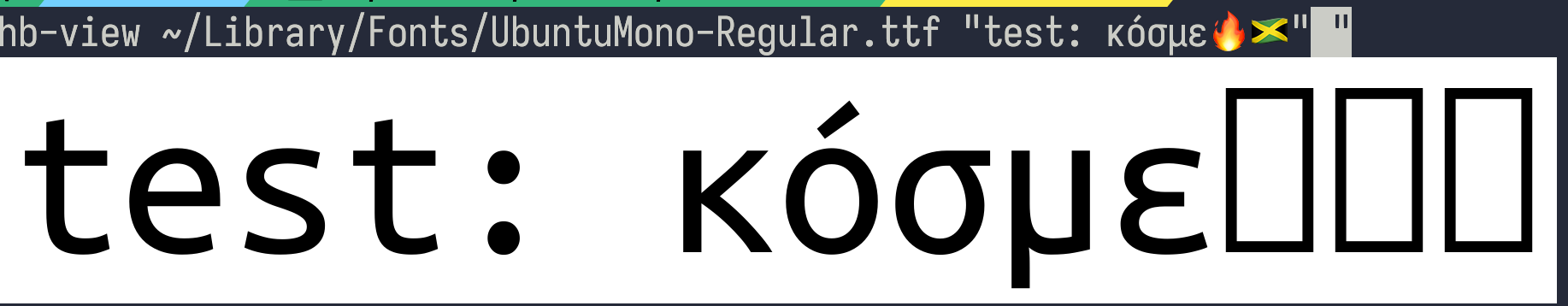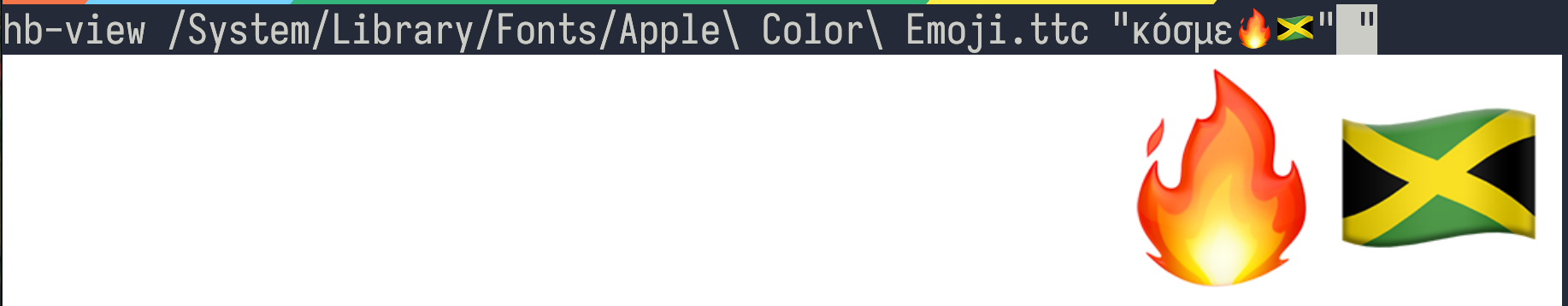# harfbuzz

Jul 13, 2023

Harfbuzz has some convenient command-line utilities to expose its internals. Here, we'll ask it to tell us how it shapes a string with some ascii characters followed by unicode characters and an emoji, in a particular font (UbuntuMono-Regular in this case):

$hb-shape ~/Library/Fonts/UbuntuMono-Regular.ttf "test: κόσμε🔥" [t=0+500|e=1+500|s=2+500|t=3+500|colon=4+500|space=5+500|kappa=6+500|uni1F79=7+500|sigma=8+500|uni03BC=9+500|epsilon=10+500|.notdef=11+500]  The default output syntax reports each glyph name (or glyph index if there is no name) followed by its cluster value, its horizontal and vertical position displacement, and its horizontal and vertical advances. It's useful to note that hb-shape tells us that the fire emoji is listed as .notdef; i.e. it was unable to shape the cluster within the given font. (I'm still working on the terminology here, so if I make mistakes, I apologize) You can use hb-view to actually render it to an image. It's neat that hb-view detects that my terminal is capable of image output, and displays an image by default:The fire emoji is a relatively easy case; it's just one character; the Jamaican flag is actually two characters. $ hb-shape ~/Library/Fonts/UbuntuMono-Regular.ttf "test: κόσμε🔥🇯🇲"
[t=0+500|e=1+500|s=2+500|t=3+500|colon=4+500|space=5+500|kappa=6+500|uni1F79=7+500|sigma=8+500|uni03BC=9+500|epsilon=10+500|.notdef=11+500|.notdef=12+500|.notdef=12+500]


In fact, just entering this string messes up my terminal's font rendering! (issue here)

We can see from the hb-shape output that we will get three tofu blocks when we render the string; it ends with three .notdef, which are our two grapheme clusters.

$hb-view ~/Library/Fonts/UbuntuMono-Regular.ttf "test: κόσμε🔥🇯🇲"(You can see the artifacts at the end of the terminal line there) Anyway, we can render our emoji with the apple emoji font, but of course we'll lose the characters: $ hb-view /System/Library/Fonts/Apple\ Color\ Emoji.ttc "κόσμε🔥🇯🇲"Some documentation for chromium talks about how they handle font fallback:

The section Text Shaping illustrates that font selection during shaping is part of an iterative process, which first tries to use as many glyphs as possible from the primary font, then in subsequent iterations proceeds to fill gaps from the secondary font and so on until there are no more so called .notdef glyphs, i.e. no more boxes of text for which no glyph was found.

\$ hb-shape /System/Library/Fonts/Apple\ Color\ Emoji.ttc "κόσμε🔥🇯🇲"
[.notdef=0+800|.notdef=1+800|.notdef=2+800|.notdef=3+800|.notdef=4+800|u1F525=5+800|u1F1EF_u1F1F2=6+800]


This time, we can see that harfbuzz is able to shape the emoji in the apple emoji font, but not the letters.

↑ up